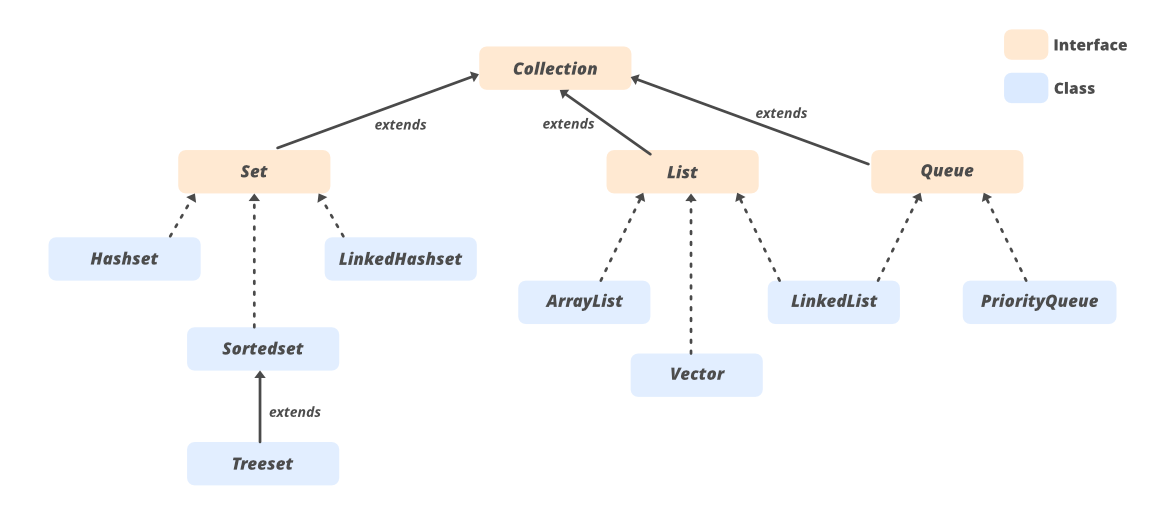# Implementing Sorted Vector in Java

• Last Updated : 20 Nov, 2020

Vector is a class that implements the List interface. It is a type of dynamic array that means the size of a vector can be grown or shrink during the execution of the program. The initial size of the vector is 10 and if we insert more than 10 elements then the size of the vector is increased by 100%, or we can say that it doubles the size. Like, initially the size of the vector is 10, and if we insert more than 10 elements the size of the vector is 20 and after inserting 20 elements in the vector, the size of the vector will be 40.

The Following diagram of the collection API

In which there is an Interface name Collection which is extended by Set, List, and Queue interfaces, and List interface is extended by ‘Vector’ class.Approaches: Implementation for Descending Order Sorting

For Implementing SortedVector

1. Extend the Vector. (Demonstrated here)
2. Extend ArrayList.
4. Directly implement the List interface.

Algorithm:

1. Extend vector class for implementation of SortedVector.
2. A private data member for storing data in SortedVector.
3. For creating objects of SortedVector, we have to define all constructors.
2. If the size of the data member is less than or equal to 1 then there is no need for sorting.
3. If the size is greater than 1 then sort it.
4. If the user entered strings then raising an error.
5. In the end, add elements in a sorted vector.
6. Clear SortedVector and after that add all data in sorted order.

Below is the implementation of the above approach:

## Java

 `// We are going to implement sorted vector``// from inbuilt Vector class.``import` `java.util.*;`` ` `class` `SortedVector ``extends` `Vector
Output
```After 1nd element Insertion:
After 1st element Insertion:
1
After 2nd element Insertion:
99
1
After 3rd element Insertion:
99
2
1
After 4th element Insertion:
99
2
1
0
```

Time Complexity: O(n2 log n)

My Personal Notes arrow_drop_up